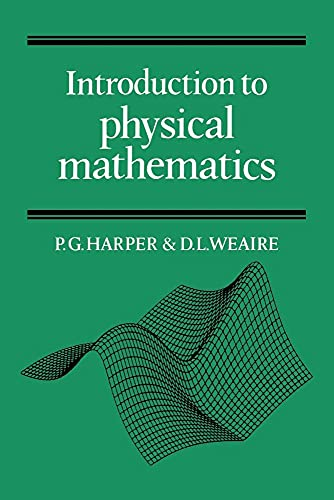# Introduction to Physical Mathematics by P. G. Harper

In Stock
\$16.99
+10% GST
Directed primarily at college and university undergraduates, this book covers at basic level the essential applications of mathematics to the physical sciences. It contains all the usual topics covered in a first-year course such as vectors, matrices, differential equations, basic mathematical functions and their analysis, and power series.
Only 4 left

## Introduction to Physical Mathematics Summary

### Introduction to Physical Mathematics by P. G. Harper

Directed primarily at college and university undergraduates, this book covers at basic level the essential applications of mathematics to the physical sciences. It contains all the usual topics covered in a first-year course such as vectors, matrices, differential equations, basic mathematical functions and their analysis, and power series. There is a strong emphasis on qualitative understanding (such as curve sketching) and practical methods of solution. The latter take due account of the impact of computers on the subject. The principles of mathematical expression are illustrated by copious examples taken from a wide range of topics in physics and chemistry. Each of the short chapters concludes with a summary and a large number of problems.

### Why buy from World of BooksOur excellent value books literally don't cost the earthFree delivery in AustraliaEvery used book bought is one saved from landfill

1. Introduction; 2. Errors; 3. Cartesian coordinates; 4. Vectors; 5. The scalar product; 6. The vector product and rotation; 7. Matrices in physics; 8. The transformation of matrices; 9. The matrix eigenvalue equation; 10. Exponential and logarithm functions; 11. Sine and cosine functions; 12. Graph plotting and curve sketching; 13. Differentiation; 14. Approximations; 15. Power series and Taylor's expansion; 16. Partial differentiation; 17. Integration; 18. The differential equation; 19. Solving first-order differential equations; 20. Second-order differential equations; 21. Solving second-order differential equations; 22. The complex exponential; 23. The circuit equation; 24. Harmonics and Fourier series; 25. The diffusion equation; 26. Waves; 27. The rate of change of a vector; 28. The scalar field and gradient operator; 29. The vector field; 30. Line integration; 31. The potential field; 32. Surface and volume integration; 33. Flux and divergence; 34. Circulation and the curl; 35. Conclusion; 36. Miscellaneous exercises.

GOR003595416
Introduction to Physical Mathematics by P. G. Harper
P. G. Harper
Used - Very Good
Paperback
Cambridge University Press
1985-03-07
276
0521269083
9780521269087
N/A
Book picture is for illustrative purposes only, actual binding, cover or edition may vary.
This is a used book - there is no escaping the fact it has been read by someone else and it will show signs of wear and previous use. Overall we expect it to be in very good condition, but if you are not entirely satisfied please get in touch with us.